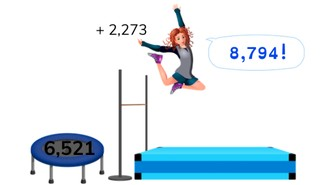# Addition to 10,000 without regrouping

Students learn to add to 10,000 without regrouping.8,000 schools use Gynzy92,000 teachers use Gynzy1,600,000 students use Gynzy

## General

The students learn to count to 10,000 without regrouping.

## Standards

CCSS.Math.Content.4.NBT.B.4

## Learning objective

Students will be able to add to 10,000 without regrouping.

## Introduction

Have the students solve the three jumping problems.

## Instruction

Briefly recall the concepts of thousands numbers, hundreds numbers, tens numbers and ones values. Then you explain how you can add to 10,000 without regrouping. Explain that you first see how many cubes of 1,000 you have. Then you add them together (2,000). Next, you add the sheets of hundreds numbers together (200 + 400 = 600). Now you add up the tens numbers (30 + 10 = 40). Finally, you count the ones values (0). Now you add all the numbers together: 2,000 + 600 + 40 + 0 = 2640. Practice the next problem together with the students and fill it in. Have the students share out loud how they solved the problem. The students answer the next few problems on their own. Then you repeat the steps to a simple adding problem. Next, you practice another problem together with the students. The students should solve the next few problems independently. For the story problem, first have the students take the problem from the story. Then they add the thousands numbers, the hundreds numbers, the tens numbers and the ones values together. Then have the students solve two more story problems on their own.

Check if the students can add to 10,000 without regrouping by asking the following questions:
- How do you solve a problem like this one (4,253 + 1,145 = ) in steps?
- What steps do you use to do this?

## Quiz

The students first practice addition to 10,000 without regrouping using a visual aid. For the next assignment they just get the number problem. The third assignment is a story problem.

## Closing

Discuss the relevance of being able to add to 10,000 without regrouping with the students. As a closing activity you can have the students make their own jumping problems, by dragging a trampoline and a figure to the section on the right and then solving the problem.

## Teaching tips

Students that have difficulty adding to 10,000 without regrouping can first practice adding numbers in jumps of 10, 100, and 1,000 to 10,000. Then have them add a number to this, for example 1,200, with the correct jumps.

## Instruction materials

MAB blocks

### The online teaching platform for interactive whiteboards and displays in schools

• Save time building lessons

• Manage the classroom more efficiently

• Increase student engagement